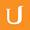## Equation of the Regression Line - Intro to Machine Learning

Title:
Equation of the Regression Line - Intro to Machine Learning
Description:

06-19 Equation_of_the_Regression_Line

more » « less
Video Language:
English
Team:Udacity
Project:
ud120 - Intro to Machine Learning
Duration:
0:42Udacity Robot edited Chinese, Simplified subtitles for Equation of the Regression Line - Intro to Machine LearningUdacity Robot edited Chinese, Simplified subtitles for Equation of the Regression Line - Intro to Machine LearningUdacity Robot edited Chinese, Simplified subtitles for Equation of the Regression Line - Intro to Machine LearningUdacity Robot edited Portuguese, Brazilian subtitles for Equation of the Regression Line - Intro to Machine LearningUdacity Robot edited Portuguese, Brazilian subtitles for Equation of the Regression Line - Intro to Machine LearningUdacity Robot edited Arabic subtitles for Equation of the Regression Line - Intro to Machine LearningUdacity Robot edited Arabic subtitles for Equation of the Regression Line - Intro to Machine LearningUdacity Robot edited Arabic subtitles for Equation of the Regression Line - Intro to Machine Learning